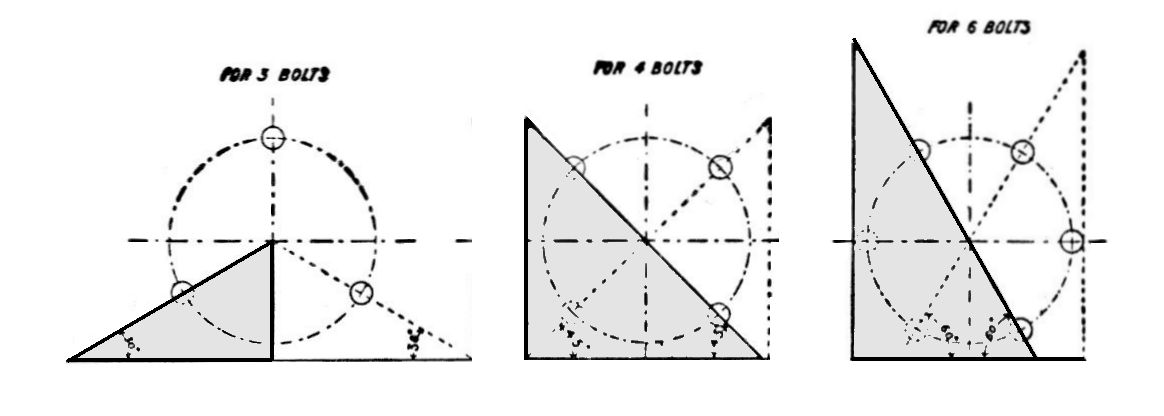## USE OF SET SQUARES TO DIVIDE A CIRCLE INTO A NUMBER OF EQUAL PARTS.

To mark off the bolt holes in a flange the set squares can be used for a flange having 3 or 6 holes, the 60° set square is used and for flange having 4 or 8 holes, the 45° set square. To divide circle into 5 equal spaces for a flange having 5 holes, proceed as shown for the construction of polygon.

To subdivide into a greater number of spaces other than mentioned, dividers or compasses are used to bisect one of the angles obtained by use of set squares and the subdivision marked in all other spaces.In many cases where the set squares cannot be employed to divide up circle, a protractor may be used, e.g., if a flange has to have 7 or 9 holes, 360° / 7 = 51 3/7° 360° / 9 = 40°, gives the angle between the centre lines of adjacent holes. Odd numbers of bolts in flanges are seldom found as it is more convenient to go to the next even figure.

For large flanges such as in cylinder covers, the circumference of pitch circle is calculated, and by dividing by the number of holes required in flange the circumferential pitch is obtained, this pitch is then stepped round the circle, using dividers.

NOTE-The dividers will be set to an amount a little less than this pitch as they step the chord and not the arc or circumferential pitch.